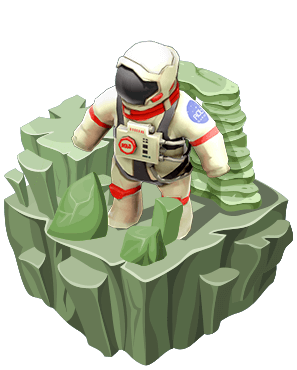# Coding Adventure 7: Clues to aliens messageLet's go to the moon, back and forth

learn about advanced loops, arrays and functions while you launch a rocket to the moon

## 描述

Solve these equations to uncover the alien message. Can you do it?

## Alien's changing equations!

Solve the equation and write the answer on the yellow cell. The numbers change and the operator can be either plus or minus. When you get the correct answer, the first letter of Alien's message will be revealed.

## Tricky equations

Solve the equation and write the answer on the yellow cell. The numbers change and the operator can be either plus, minus, or multiply.  Once you get the correct answer, another letter will be revealed.

## Solve the equation

Solve the equation and write the answer on the yellow cell. The numbers change and the operator can be either plus, minus, multiply, or divide. Can you solve this to reveal the third letter?

## Three variables

For the fourth letter, Robo must solve this three variable equation and write the answer on the yellow cell. Be careful, the numbers change.隱私政策使 使用條款